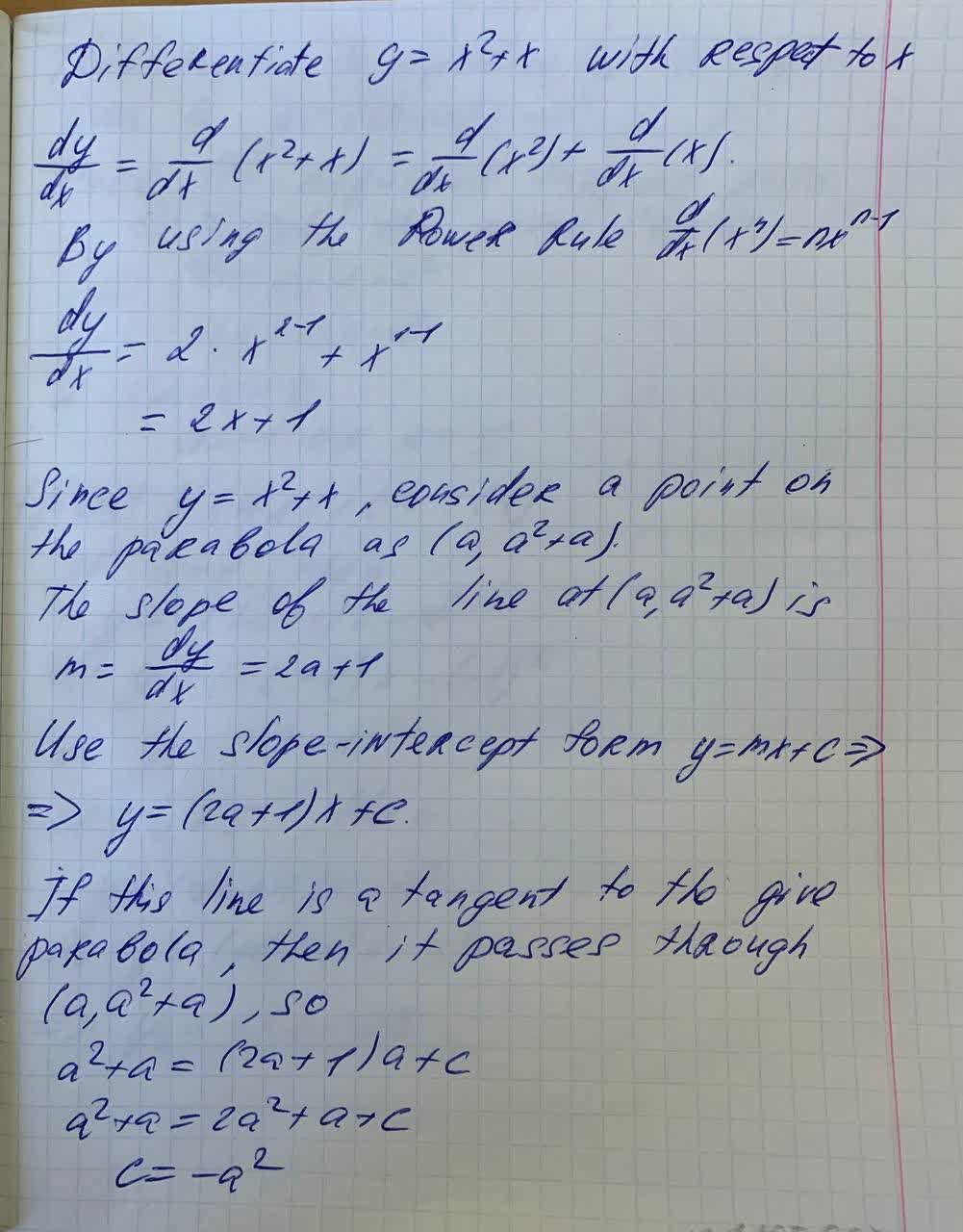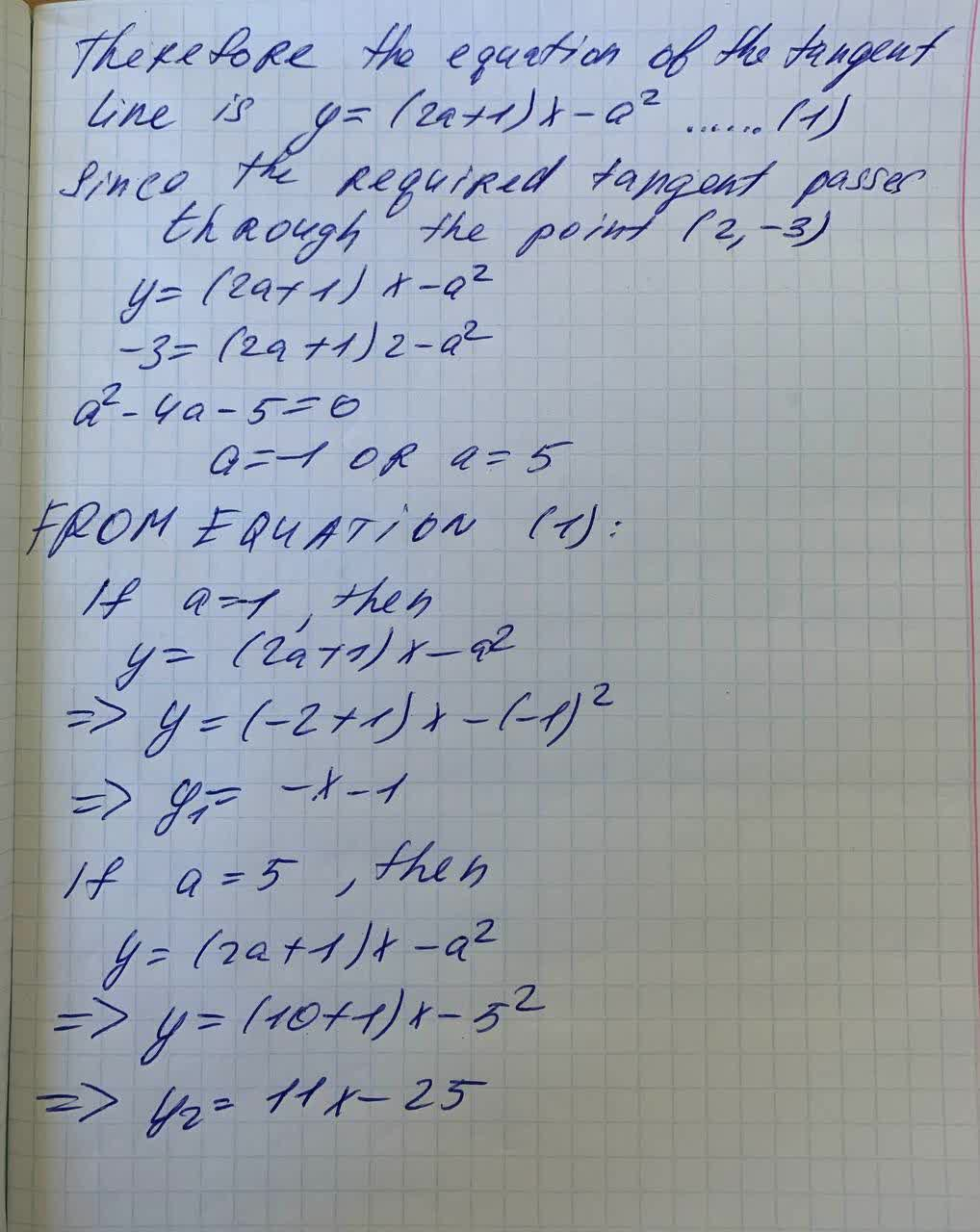# Find equations of both lines through the point (2, ?3) that are tangent to the parabola PSSinead Mcgee 2021-09-24 Answered
Find equations of both lines through the point (2, ?3) that are tangent to the parabola $$\displaystyle{y}={x}^{{2}}+{x}$$.
$$\displaystyle{y}_{{1}}$$=(smaller slope quation)
$$\displaystyle{y}_{{2}}$$=(larger slope equation)

• Questions are typically answered in as fast as 30 minutes

### Plainmath recommends

• Get a detailed answer even on the hardest topics.
• Ask an expert for a step-by-step guidance to learn to do it yourself.broliY
Calculation: